Back to index of science and math pages by Donald Sauter.

### Adding and Subtracting Mixed Numbers - almost mindless!

What teachers and textbooks put students through in adding and subtracting mixed numbers is a math crime, in my view. They've got numbers and symbols going every which way in some sort of deranged mathematical crossword puzzle. For a couple of examples:

```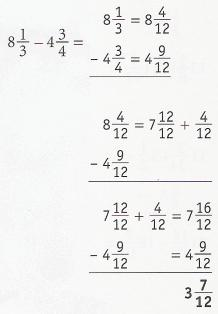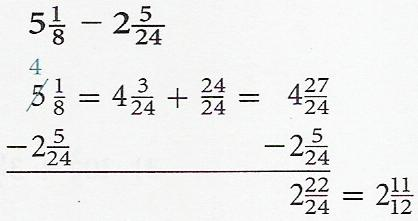```

It had never occurred to me that my simple, straightforward method of doing this was in any way radical, but I was tutoring a college student once, and when the math department saw how she was adding and subtracting mixed numbers, all heck broke loose.

"You can't do it like that!"

"That won't always work!"

"I tell you, I wouldn't trust her as a nurse!"

The last from the head of the math department when I stormed into his office . . .

So I guess I must have something really, really good. Time to share it with the world.

Here are some benefits of my method. It works perfectly well for any number of mixed numbers being added or subtracted. You never have to borrow, or even think about whether a bigger fraction is being subtracted from a smaller fraction. The solution proceeds across the page, left to right, as all expressions should be evaluated or simplified. (This is in distinction to solving equations, which go down the page step by step.) I'm a stickler for form in math problems. It makes things that much simpler, more secure, and more understandable.

The procedure can be distilled down to a series of steps that are either mindless or almost so. Here's the "recipe" for adding and subtracting mixed numbers. Read the list through a few times to catch the flow.

1. Equal sign.
2. Whole numbers.
3. Plus sign.
4. Fraction line.
5. LCD.
6. Inflate numerators.
7. Wrap up.

Here's a sample problem solved completely, showing all work, using the recipe.Now, here's the solution shown step by step, starting with the original problem:Step 1: Equal sign.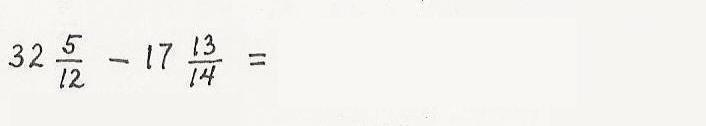That's right; all you do is slap an equal sign down. It counts as a step. Am I being ridiculous? Does anyone need to be told to do that? No; and yes, particularly in this age when mathematical form and "grammar" seem to have gone by the board.

But more to the point is that all of math is done step by step, so do the next step, no matter how trivial. Writing the equal sign gets you rolling; it builds up your momentum; you're one step closer to being done.

I often see a student look at a problem, then turn his eyes to the ceiling, apparently expecting the whole problem to fall together at once somehow, and the final answer to flash into his brain. Forget it; that's not how math works. Math is simple step by simple step.

Step 2: Whole numbers.

This means add (or subtract) the whole numbers. Don't even think about the fractions. 32 - 17 = 15 .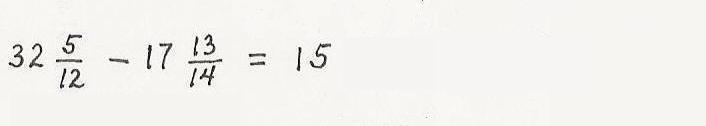Step 3: Plus sign.Another mindless step, but we're rolling! And, yes, "plus sign" means "plus sign", regardless of whether the problem involves any subtraction. Without the "+", the whole number would be seen to multiply the fraction we're about to construct. (Notice that a mixed number, formed strictly of three whole numbers, is an exception to the math convention that two entities butted up against each other imply multiplication.)

Step 4: Fraction line.And another mindless step - math is so easy! Be generous; make the fraction line long enough to accommodate a few numbers on top.

Step 5. LCD, part 1.

First of all, remember that the LCD (Least Common Denominator) is just an LCM (Least Common Multiple) that is used as a denominator. It's easier to talk about LCMs.

It's usually the case that an LCM can be found mentally very quickly and easily - assuming you know what an LCM is. And the name really says it all: an LCM is a multiple that works commonly for all the numbers in question, and is the smallest (least) such multiple.

The LCM is the smallest number that all the given numbers go into, (that is, without remainder.)

Most textbook fractions won't have you going beyond the multiplication table for the LCM. You can often nail it by going up the line of multiples of the biggest number of the set. (Don't forget that the biggest number itself might work as the LCM.)

I've chosen a couple of denominators here for which, I hope, the LCD isn't so obvious to everybody. In such a case you have to examine the factors of each denominator to construct the LCD. I don't mean to make this a complete lesson in finding LCMs, but there is really very little to it, as can be seen in this example.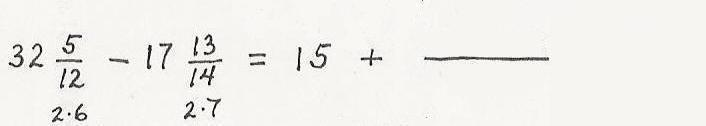Do your factoring work right under the denominators in the original problem. Understand that it's not generally necessary, or desirable, to factor each number completely. What you want to do is take the biggest common "bite" out of each number. In this case you see both numbers are even, so you pull out a 2.

For the 12, that leaves a 6; for the 14, a 7. You stop at that point because you know that 6 and 7 have nothing in common (meaning factors), even if you can do more factoring. (The 7 is prime, of course.)

So you look at your work and see you need just one of the 2s, and a 6 and a 7 to construct the LCD. 2 x 42 = 84; that's it!

Step 5: LCD, part 2.

Write the LCD under the fraction line. It's not incorrect to write separate fractions with the new denominator for each of the original fractions, but that practice bugs me to death. Besides being more writing and messier, it shows a lack of complete understanding of what a Least COMMON Denominator is all about. The one denominator will work for all the numerators. So write it once!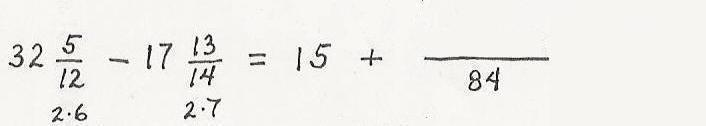I'm afraid it would look a bit radical here, but you'll see in the section of examples below that I actually recommend not multiplying the LCD factors together until you really need to. Just string them out separately in the denominator. That helps you see the "inflation factor" for each numerator (see the next step), and a factor may cancel out before you need to multiply.

Step 6: Inflate numerators.

Here, you keep in mind the factors you used to construct the LCD, which were 2, 6, and 7 in this case. You look back at the 12 and ask yourself, what did I multiply by to "inflate" 12 to 84? We don't really start from scratch; we've already done the legwork. The 12 is made up of a 2 and 6. What's missing is the 7, so that's the one. We inflated the denominator by 7 (we're talking multiplication here), so we must inflate the numerator by the same amount. 7 x 5 = 35.

The thought process is exactly the same for the second fraction. The denominator, 14, made up of the 2 and 7, was inflated by the "other" factor, 6; so we inflate the numerator by 6: 6 x 13 = 78. Write the inflated numerators above the fraction line, remembering the sign from the original problem. The minus sign distributes over the fraction part of the mixed number, of course.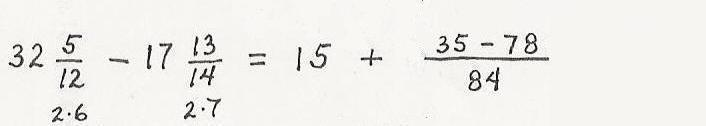Step 7: Wrap up, part 1.

Perform the additions and subtractions indicated in the numerator "off-line"; simply jot the answer just above it. We don't like moving a whole expression forward for the sake of a piddly arithmetic calculation. Unnecessary copying is a waste of time and effort and can introduce errors.In this case, you see that the 35 and 78 have opposite signs, and so are "working against" each other. Thus, the magnitudes are simply subtracted: 78-35=43. You look back and see the weightier one, 78, was negative so the difference has to be negative. If a student is the least bit shaky about adding and subtracting signed numbers, he receives an electro-shock (Zap!) and is sent back to the beginning of math. Tough love.

Step 7: Wrap up, part 2.

Now your mind tunes out the obsolete riffraff, and what you see is just

```            43
15 - ----
84
```

I go to the trouble to type it out like that because you will always write and work with perfectly horizontal fraction lines, no matter how they're written in the book. The whole idea of a fraction line is to separate what's on top from what's down below. 15 - 43/84 doesn't cut it. Why we evaluate expressions left to right is to preserve this upness and downness from one step to the next. The clunky textbook "crossword puzzle" form for adding and subtracting mixed numbers, even with horizontal fraction lines, makes a messy jumble of numerators and denominators (besides the general difficulty of applying it to more than two mixed numbers.) Expressions are evaluated left to right. End of sermon; back to wrapping up this problem.

A whole number minus a fraction is a simple, in-head problem. The unit from 14 to 15 is divided into 84 equal steps; we step back 43; and land at step 41. Thus, our final answer,

```          41
14 ----
84
```

Once again, if a student hesitates a moment on this - electro-shock (Zap!), and back to the beginning of math. One day he'll thank you for it.We're always on the lookout for a simplification of a fraction in a final answer. Here, we see instantly that 41 is prime, because we don't recognize it from our multiplication table, and it fails the tests for factors of 2, 3, or 5. (Sneaky, ol' 91 is the only two-digit number that fails those tests, and yet is not prime.) We sort of know that if there wasn't a reduction in the previous step there won't be one in the final answer, but we take a hard look at the final answer, anyhow.

And, last but not least, box your answer! It worked great in my day, and there's no reason your teacher won't love it, too.

*** Appendix 1: Examples ***

Warning! I planted some ERRORS in the examples below, thinking that might snag some visitors into taking a closer look and actually stepping through some examples. I'd be happy to add your name to a list of people who spotted all the errors.

We'll start with the two textbook examples shown at the top of the page, done their way and done my way. I've boxed the steps between the statement of the problem and the final answer to show at a glance the striking difference in the amount of work required.

In the first example, you recognize that the two denominators have nothing in common (meaning factors), in which case the LCD is simply the product of the two.

```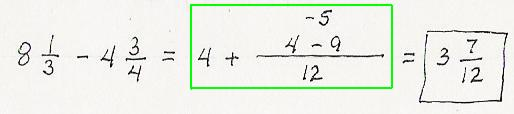```

In the second example, you immediately see that one denominator is a multiple of the other, in which case the LCD is always just the big one.

```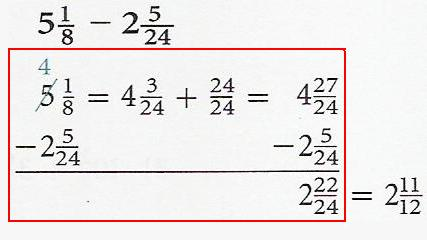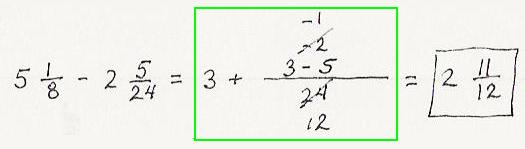```

Here's an example where a firm understanding of what an LCD is, and a quick mental look at our multiplication table, coughs the LCD right up; no diddling around with prime factors.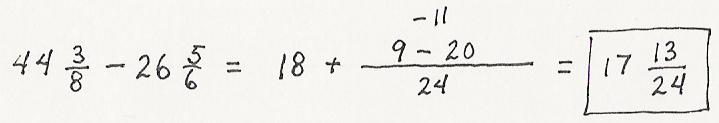Our recipe handles any number of terms with ease; here's a problem with three mixed numbers. Once again, the LCM is good, ol' 24. (Bor-ring!) He pops up a lot since he's a multiple of 2, 3, 4, 6, 8 and 12.Notice that we had a cancellation before proceeding to the final answer. That raises the question, why even bother multiplying out the LCD, if we might be dividing out one or more of the factors later on? Why not just leave the factors of the LCD separate until we need to multiply them?

Even if you're not lucky enough to get a cancellation out of the deal, you should notice how much easier it is to see the "inflation" factor for each fraction when looking at a neat line-up of the LCD factors. It's best to just leave them in the same order, left to right, that you found them. And I'll say again that we like the LCD factors in the biggest bites possible; we take them down to prime numbers only if forced to.

I urge you to try this; the advantage is probably not so obvious just looking at examples.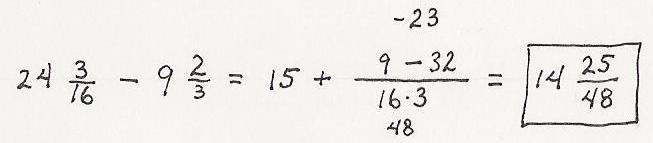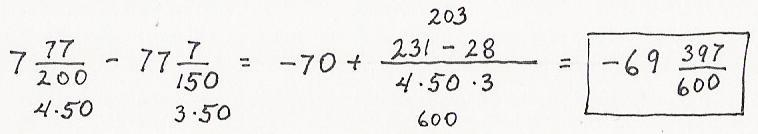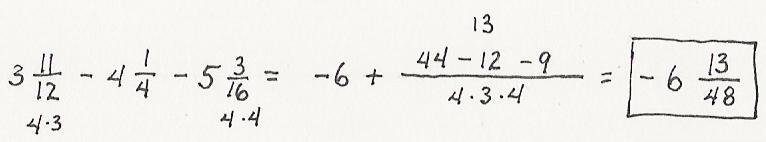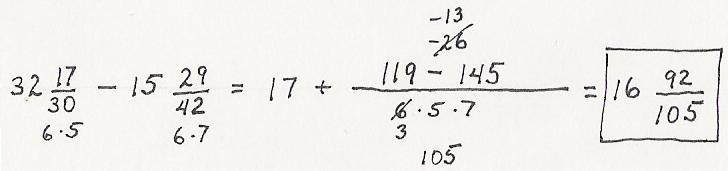There's another one with a cancellation, but it always seemed to me that this doesn't happen as often as you'd expect. Is there an explanation for this that's somewhat intuitive? When your LCD has a bunch of small factors in it, why should it be so unusual for the numerator to land on a multiple of one of them? (See Appendix 2 further down for more discussion.)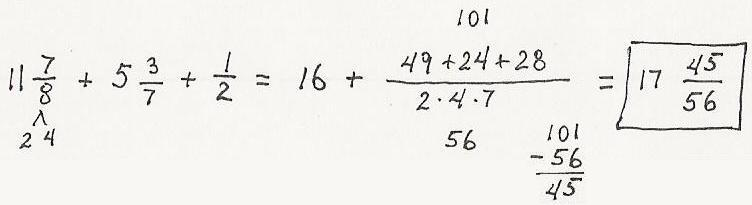The final example is from a workbook that often used unreduced fractions in the original problem. That's strange, but I'll say it keeps you on your toes. (I jumped right in finding the LCM for 64, 30, and 25 - oof!) You'd find that there is not a hard rule about whether or not to simplify first. Sometimes, as in this case, it makes things much easier to simplify first. In other cases, an unreduced fraction may actually be the inflated fraction you want, or at least a step closer. (Why take 8/10 down to 4/5 if it just has to go up to 16/20?)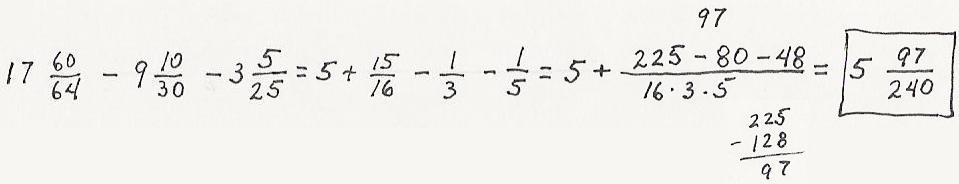Notice the off-line calculations. Keep them out of the way of the flow of the problem, and put enough care into them so that everyone can easily see what it's for.

*** Appendix 2: Where are all the reductions? ***
(A dissertation topic for you!)

I've mentioned above my perplexity about why we seem to get reductions much less often than we'd expect when adding and subtracting mixed numbers. I actually tried to work up an example where there would be a lot of satisfying cancellations before going on to the final answer.

I chose three denominators, 9, 30 and 35, which use lots of little prime factors. So, ignoring the upfront whole numbers, the problem would take the form,

```       A        B        C
--- +/- ---- +/- ----
9       30       35
```

where each fraction is proper and reduced.

The LCD, in prime factors, is 3 x 3 x 2 x 5 x 7 = 630, but the whole point was supposed to be that you really don't need to multiply it out.

After applying the appropriate inflation factors, the numerator works out to:

```      Inflated numerator = 70A +/- 21B +/- 18C    (Eq. 1)
```

I wrote a computer program that loops through all the acceptable whole numbers for A, B and C, and the associated plus/minus signs.

A = {1 2 4 5 7 8} - 6 choices
B = {1 7 11 13 17 19 23 29} - 8 choices
C = {1 2 3 4 6 8 9 11 12 13 16 17 18 19 22 23 24 26 27 29} - 20 choices

With 4 combinations of plus and minus signs, that works out to 3840=6x8x20x4 numerators calculated, and . . .

Not a single one of them was divisible by 2.

Not a single one of them was divisible by 3.

Not a single one of them was divisible by 7.

But tons of them were divisible by 5.

Why should that be??? What's so darn hard about hitting a multiple of 2 or 3? Even multiples of 7 are fairly dense out there. On the other hand, what makes multiples of 5 so ubiquitous?

Okay, if we put aside gut feeling and put on our math hat, we can explain all of that. For your convenience, here's Eq. 1 again:

```      Inflated numerator = 70A +/- 21B +/- 18C    (Eq. 1)
```

Notice that terms one and three must each be even, while term two, 21B, must be odd, so the sum must be odd. No multiples of 2.

Likewise, terms two and three must each be a multiple of 3, while term 1, 70A, can never be a multiple of 3. So the sum can never be a multiple of 3.

Likewise, terms one and two must each be a multiple of 7, while term 3, 18C, can never be a multiple of 7. So the sum can never be a multiple of 7.

Now, what about 5? Term 1, 70A, must be a multiple of 5. Neither term 2 or term 3 can be a multiple of 5, but term 2 must be odd while term 3 must be even. So it doesn't surprise us that quite often the sum of term 2 and term 3 hits a multiple of 5.

Still, very strange, to my mind. Who on earth would know by looking at the original problem, no matter what numerators are chosen, that you can't possibly get a reduction by 2, 3, or 7 at the end, but have a decent chance of getting a reduction by 5?

Is there an intuitive way to look at this? Is there a quick test of the LCD factors that will show which ones don't have a chance of dividing out?

I suppose I could play around with it a little more, but that's enough for one web page.

Contact Donald Sauter: send an email; view guestbook; sign guestbook.
Back to Donald Sauter's main page.
Rather shop than think? Please visit My Little Shop of Rare and Precious Commodities.
Back to the top of this page.

Helpful keywords not in the main text: addition and subtraction of mixed numbers; mathematics.

Parents, if you're considering tutoring or supplemental education for your child, you may be interested in my observations on Kumon.

